# Map 3

Map scale is M = 1: 25000 . Two cottages which are shown on the map are actually 15 km away. What is its distance on the map?

x =  600 mm

### Step-by-step explanation: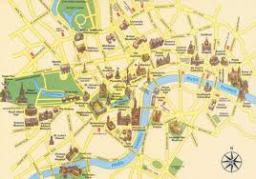We will be pleased if You send us any improvements to this math problem. Thank you!Tips to related online calculators
Check out our ratio calculator.
Do you want to convert length units?

## Related math problems and questions:

• The scaleThe distance of 15 cm on the map corresponds to a distance of 27 km in reality. What is the scale of the map?
• Scale of the map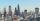The distance between two cities is actually 30 km and the map is 6 cm. What is the scale of the map?
• Map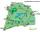On the tourist map at a scale of 1 : 50000 is distance between two points along a straight road 3.7 cm. How long travels this distance on a bike at 30 km/h? Time express in minutes.
• What scale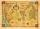What scale is the map drawn if it shows a 15km route from the station to the ruins with a line 30cm long? A) 1: 2000 B) 1: 5000 C) 1: 20,000 D) 1: 50,000
• Scale of the mapDetermine the scale of the map if the actual distance between A and B is 720 km and distance on the map is 20 cm.
• Scale of the mapDetermine the map's scale, which is the actual distance of 120 km l represented by a segment long 6 cm.
• Map 2At what scale is made map if the distance 8.2 km corresponds on the map segment 5 cm long?
• Two villages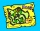On the map with a scale of 1:40000 are drawn two villages actually 16 km away. What is their distance on the map?
• Two monuments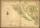The distance between two historical monuments on a map with a scale of 1: 500000 is 48 mm. Find the actual distance between these monuments in km.
• Two villages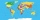Two villages are 11 km and 500 m away. On the map, their distance is determined by a 5 cm long line. Find the scale of the map.
• ScaleThe swimming pool is long 110 m and 30 m wide. The plan of the city is shown as a rectangle with an area 8.25 cm2. What scale is the city plan?
• Map scaleOn a 1:1000 scale map is a rectangular land of 4.2 cm and 5.8 cm. What is the area of this land in square meters?
• Map scaleGarden has on plan on a scale of 1: 150 width 22 cm and length 35 cm. What is the real area of the garden?
• Map scaleThe rectangular plot has a scale of 1: 10000 area 3 cm2 on the map. What content does this plot have on a 1:5000 scale map?
• On the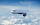On the map of Europe made at 1: 4000000, Bratislava and Paris' distance is 28 cm. At what time an airplane flying 800 km/h will fly this journey?
• The distance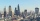The distance between two towns on a given map is 3 ½ cm. If ½ cm represents 6km. , what is the distance between the two towns?
• The plan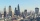The plan of the housing estate is in three scales 1: 5000,1: 10000,1: 15000. The distance between two points on a plan with a scale of 1: 10000 is 12 cm. What is this distance on the other two plans? What is this distance?